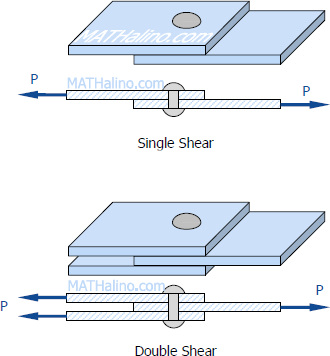# Shear Stress

Forces parallel to the area resisting the force cause shearing stress. It differs to tensile and compressive stresses, which are caused by forces perpendicular to the area on which they act. Shearing stress is also known as tangential stress.

$\tau = \dfrac{V}{A}$

where V is the resultant shearing force which passes through the centroid of the area A being sheared.Subscribe to MATHalino.com on